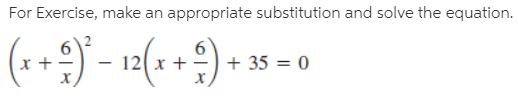# For Exercise, make an appropriate substitution and solve the equation. (++2) - 12(*+9)* + 35 = 0

Questionhelp_outlineImage TranscriptioncloseFor Exercise, make an appropriate substitution and solve the equation. (++2) - 12(*+9)* + 35 = 0 fullscreen